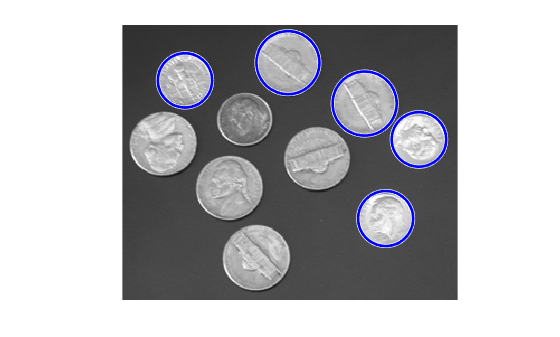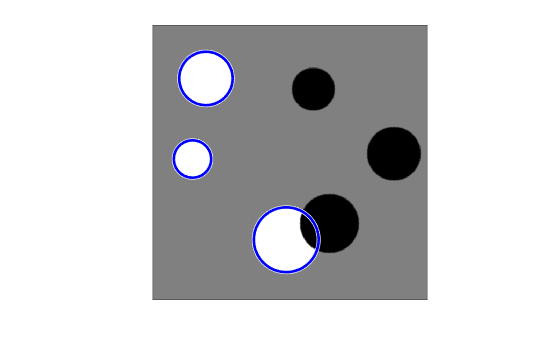Documentation

### This is machine translation

Mouseover text to see original. Click the button below to return to the English version of the page.

To view all translated materials including this page, select Country from the country navigator on the bottom of this page.

# imfindcircles

Find circles using circular Hough transform

## Syntax

``centers = imfindcircles(A,radius)``
``````[centers,radii] = imfindcircles(A,radiusRange)``````
``````[centers,radii,metric] = imfindcircles(A,radiusRange)``````
``````[___] = imfindcircles(___,Name,Value)``````

## Description

````centers = imfindcircles(A,radius)` finds the circles in image `A` whose radii are approximately equal to `radius`. The output, `centers`, is a two-column matrix containing the x,y coordinates of the circles centers in the image.```
``````[centers,radii] = imfindcircles(A,radiusRange)``` finds circles with radii in the range specified by `radiusRange`. The additional output argument, `radii`, contains the estimated radii corresponding to each circle center in `centers`.```

example

``````[centers,radii,metric] = imfindcircles(A,radiusRange)``` also returns a column vector, `metric`, containing the magnitudes of the accumulator array peaks for each circle (in descending order). The rows of `centers` and `radii` correspond to the rows of `metric`.```

example

``````[___] = imfindcircles(___,Name,Value)``` specifies additional options with one or more `Name,Value` pair arguments, using any of the previous syntaxes.```

## Examples

collapse all

This example shows how to find all circles in an image, and how to retain and display the strongest circles.

Read a grayscale image into the workspace and display it.

```A = imread('coins.png'); imshow(A)```Find all the circles with radius `r` pixels in the range [15, 30].

`[centers, radii, metric] = imfindcircles(A,[15 30]);`

Retain the five strongest circles according to the metric values.

```centersStrong5 = centers(1:5,:); radiiStrong5 = radii(1:5); metricStrong5 = metric(1:5);```

Draw the five strongest circle perimeters over the original image.

`viscircles(centersStrong5, radiiStrong5,'EdgeColor','b');`Read the image into the workspace and display it.

```A = imread('circlesBrightDark.png'); imshow(A)```Define the radius range.

```Rmin = 30; Rmax = 65;```

Find all the bright circles in the image within the radius range.

`[centersBright, radiiBright] = imfindcircles(A,[Rmin Rmax],'ObjectPolarity','bright');`

Find all the dark circles in the image within the radius range.

`[centersDark, radiiDark] = imfindcircles(A,[Rmin Rmax],'ObjectPolarity','dark');`

Draw blue lines around the edges of the bright circles.

`viscircles(centersBright, radiiBright,'Color','b');`Draw red dashed lines around the edges of the dark circles.

`viscircles(centersDark, radiiDark,'LineStyle','--');`## Input Arguments

collapse all

Input image is the image in which to detect circular objects, specified as a grayscale, truecolor, or binary image.

Data Types: `single` | `double` | `int16` | `uint8` | `uint16` | `logical`

Circle radius is the approximate radius of the circular objects you want to detect, specified as a scalar of any numeric type.

Data Types: `single` | `double` | `int8` | `int16` | `int32` | `int64` | `uint8` | `uint16` | `uint32` | `uint64`

Range of radii for the circular objects you want to detect, specified as a 2-element vector of integers of the form `[rmin rmax]`.

Data Types: `single` | `double` | `int8` | `int16` | `int32` | `int64` | `uint8` | `uint16` | `uint32` | `uint64`

### Name-Value Pair Arguments

Specify optional comma-separated pairs of `Name,Value` arguments. `Name` is the argument name and `Value` is the corresponding value. `Name` must appear inside quotes. You can specify several name and value pair arguments in any order as `Name1,Value1,...,NameN,ValueN`.

Example: `'ObjectPolarity','bright'` specifies bright circular objects on a dark background.

Object polarity indicates whether the circular objects are brighter or darker than the background, specified as the comma-separated pair consisting of `'ObjectPolarity'` and either of the values in the following table.

 `'bright'` The circular objects are brighter than the background. `'dark'` The circular objects are darker than the background.

Computation method is the technique used to compute the accumulator array, specified as the comma-separated pair consisting of `'Method'` and either of the values in the following table.

 `'PhaseCode'` Atherton and Kerbyson's  phase coding method . This is the default. `'TwoStage'` The method used in two-stage circular Hough transform , .

Example: `'Method','PhaseCode'` specifies the Atherton and Kerbyson's phase coding method.

Sensitivity factor is the sensitivity for the circular Hough transform accumulator array, specified as the comma-separated pair consisting of `'Sensitivity'` and a number in the range [0,1]. As you increase the sensitivity factor, `imfindcircles` detects more circular objects, including weak and partially obscured circles. Higher sensitivity values also increase the risk of false detection.

Edge gradient threshold sets the gradient threshold for determining edge pixels in the image, specified as the comma-separated pair consisting of `'EdgeThreshold'` and a number in the range [0,1]. Specify `0` to set the threshold to zero-gradient magnitude. Specify `1` to set the threshold to the maximum gradient magnitude. `imfindcircles` detects more circular objects (with both weak and strong edges) when you set the threshold to a lower value. It detects fewer circles with weak edges as you increase the value of the threshold. By default, `imfindcircles` chooses the edge gradient threshold automatically using the function `graythresh`.

Example: `'EdgeThreshold',0.5` sets the edge gradient threshold to `0.5`.

## Output Arguments

collapse all

Coordinates of the circle centers, returned as a `P`-by-`2` matrix containing the x-coordinates of the circle centers in the first column and the y-coordinates in the second column. The number of rows, `P`, is the number of circles detected. `centers` is sorted based on the strength of the circles.

The estimated radii for the circle centers, returned as a column vector. The radius value at `radii(j)` corresponds to the circle centered at `centers(j,:)`.

Circle strengths is the relative strengths for the circle centers, returned as a vector. The value at `metric(j)` corresponds to the circle with radius `radii(j)` centered at `centers(j,:)`.

## Tips

• Specify a relatively small `radiusRange` for better accuracy. A good rule of thumb is to choose `radiusRange` such that `rmax < 3*rmin` and ```(rmax-rmin) < 100```.

• The accuracy of `imfindcircles` is limited when the value of `radius` (or `rmin`) is less than or equal to 5.

• The radius estimation step is typically faster if you use the (default) `'PhaseCode'` method instead of `'TwoStage'`.

• Both computation methods, `'PhaseCode'` and `'TwoStage'` are limited in their ability to detect concentric circles. The results for concentric circles can vary depending on the input image.

• `imfindcircles` does not find circles with centers outside the domain of the image.

• `imfindcircles` preprocesses binary (logical) images to improve the accuracy of the result. It converts truecolor images to grayscale using the function `rgb2gray` before processing them.

## Algorithms

`imfindcircles` uses a Circular Hough Transform (CHT) based algorithm for finding circles in images. This approach is used because of its robustness in the presence of noise, occlusion and varying illumination.

The CHT is not a rigorously specified algorithm, rather there are a number of different approaches that can be taken in its implementation. However, by and large, there are three essential steps which are common to all.

1. Accumulator Array Computation

Foreground pixels of high gradient are designated as being candidate pixels and are allowed to cast ‘votes’ in the accumulator array. In a classical CHT implementation, the candidate pixels vote in pattern around them that forms a full circle of a fixed radius. Figure 1a shows an example of a candidate pixel lying on an actual circle (solid circle) and the classical CHT voting pattern (dashed circles) for the candidate pixel.

Classical CHT Voting Pattern2. Center Estimation

The votes of candidate pixels belonging to an image circle tend to accumulate at the accumulator array bin corresponding to the circle’s center. Therefore, the circle centers are estimated by detecting the peaks in the accumulator array. Figure 1b shows an example of the candidate pixels (solid dots) lying on an actual circle (solid circle), and their voting patterns (dashed circles) which coincide at the center of the actual circle.

If the same accumulator array is used for more than one radius value, as is commonly done in CHT algorithms, radii of the detected circles have to be estimated as a separate step.

`imfindcircles` provides two algorithms for finding circles in images: Phase-Coding (default) and Two-Stage. Both share some common computational steps, but each has its own unique aspects as well.

The common computational features shared by both algorithms are as follow:

• Use of 2-D Accumulator Array

The classical Hough Transform requires a 3-D array for storing votes for multiple radii, which results in large storage requirements and long processing times. Both the Phase-Coding and Two-Stage methods solve this problem by using a single 2-D accumulator array for all the radii. Although this approach requires an additional step of radius estimation, the overall computational load is typically lower, especially when working over large radius range. This is a widely adopted practice in modern CHT implementations.

• Use of Edge Pixels

Overall memory requirements and speed is strongly governed by the number of candidate pixels. To limit their number, the gradient magnitude of the input image is threshold so that only pixels of high gradient are included in tallying votes.

• Use of Edge Orientation Information

Another way to optimize performance is to restrict the number of bins available to candidate pixels. This is accomplished by utilizing locally available edge information to only permit voting in a limited interval along direction of the gradient (Figure 2).

Voting Mode: Multiple Radii, Along Direction of Gradientrmin Minimum search radius rmax Maximum search radius ractual Radius of the circle that the candidate pixel belongs to cmin Center of the circle of radius rmin cmax Center of the circle of radius rmax cactual Center of the circle of radius ractual

The two CHT methods employed by function `imfindcircles` fundamentally differ in the manner by which the circle radii are computed.

• Two-Stage

Radii are explicitly estimated utilizing the estimated circle centers along with image information. The technique is based on computing radial histograms  .

• Phase-Coding

The key idea in Phase Coding  is the use of complex values in the accumulator array with the radius information encoded in the phase of the array entries. The votes cast by the edge pixels contain information not only about the possible center locations but also about the radius of the circle associated with the center location. Unlike the Two-Stage method where radius has to be estimated explicitly using radial histograms, in Phase Coding the radius can be estimated by simply decoding the phase information from the estimated center location in the accumulator array.

## Compatibility Considerations

expand all

Behavior changed in R2019a

 T.J Atherton, D.J. Kerbyson. "Size invariant circle detection." Image and Vision Computing. Volume 17, Number 11, 1999, pp. 795-803.

 H.K Yuen, .J. Princen, J. Illingworth, and J. Kittler. "Comparative study of Hough transform methods for circle finding." Image and Vision Computing. Volume 8, Number 1, 1990, pp. 71–77.

 E.R. Davies, Machine Vision: Theory, Algorithms, Practicalities. Chapter 10. 3rd Edition. Morgan Kauffman Publishers, 2005,